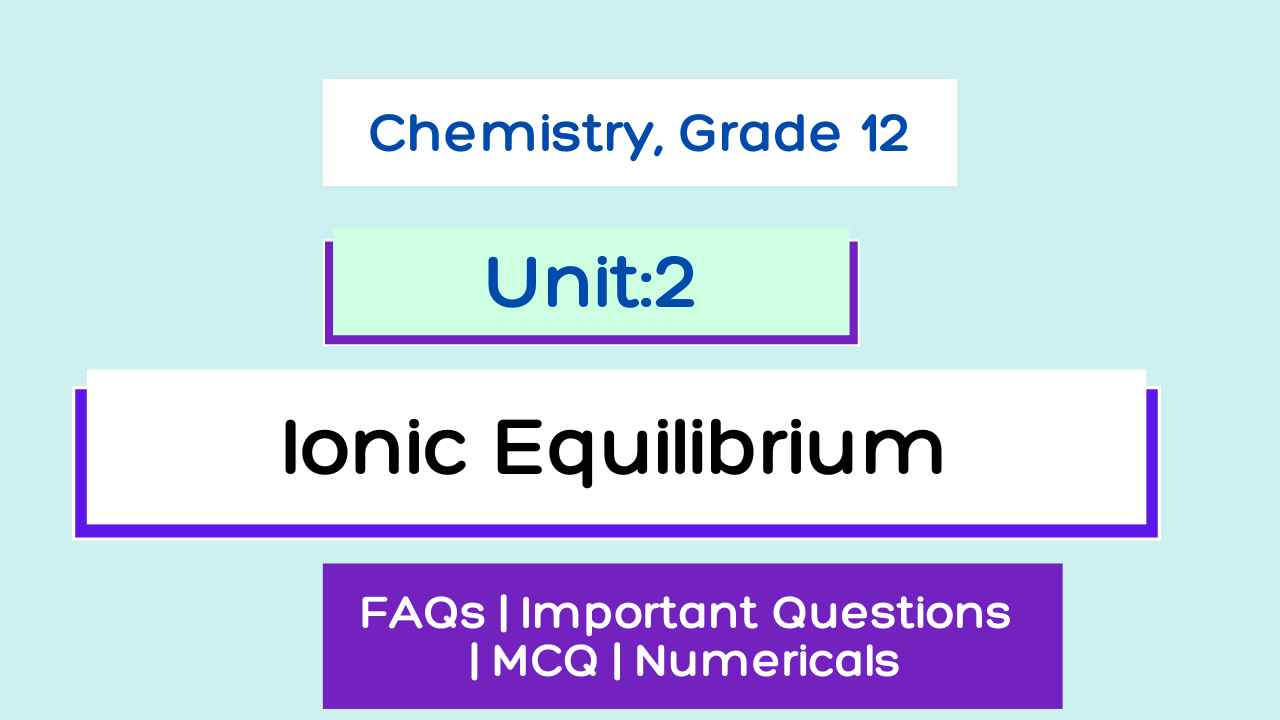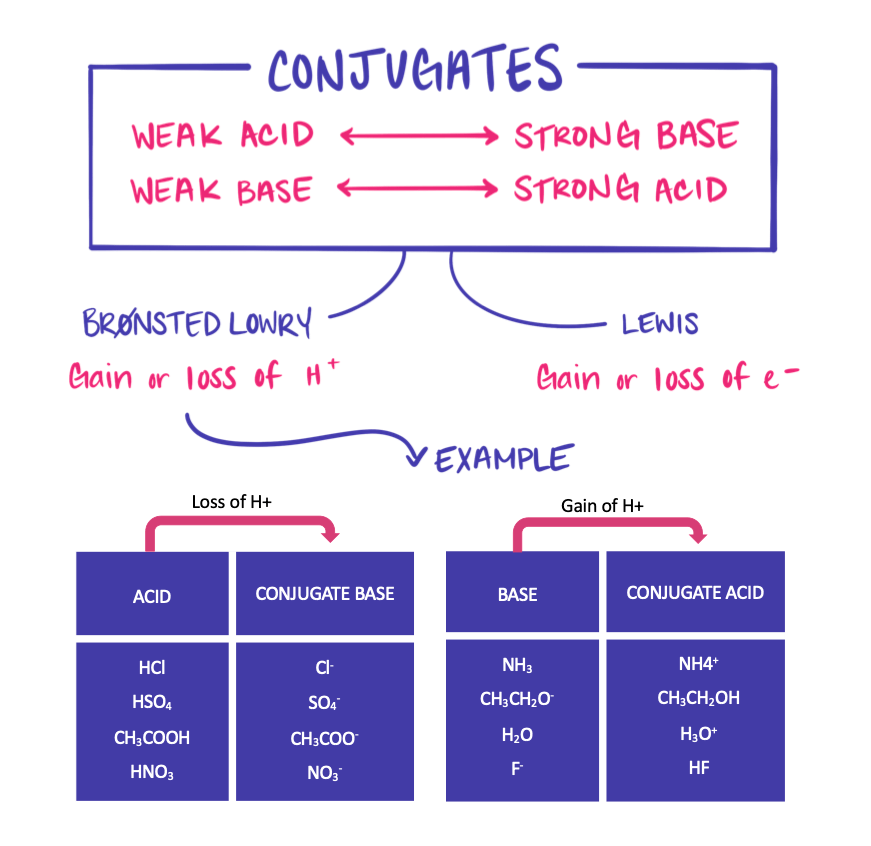# Ionic Equilibrium Class 12 Chemistry Notes

Ionic Equilibrium Notes. Complete NEB Chemistry 2080 Exercise, Question Answers, MCQs, Numerical Note of Class 12 Chemistry Unit 2, Ionic Equilibrium.

## Unit 2: Ionic Equilibrium

Complete Exercise note of Ionic Equilibrium of unit 2 Class 12 Chemistry for 2080.

Unit 2: Ionic Equilibrium Course Content
2.1 Explain the limitations of Arrhenius's concepts of acid and bases.
2.2 Define Bronsted and Lowry's concepts for acids and bases.
2.3 Define conjugate acids and conjugate bases.
2.4 Identify conjugate acid-base pairs of Bronsted acid and base
2.5 Define and explain Lewis acids and bases.
2.6 Use the extent of ionization and dissociation constant of acid (Ka) and base (Kb)
2.7 Explain the ionization constant of water and calculate pH and pOH in an aqueous medium using Kw values;
2.8 Show understanding of, and use, the concept of solubility product Ksp.
2.9 Calculate Ksp from concentrations and vice versa.
2.10 Show understanding of the common ion effect.
2.11 Describe the application of the solubility product principle and common ion effect in precipitation reactions.
2.12 Define a Buffer and show with equations how a Buffer system works.
2.13 Explain the choice of suitable indicators for acid-base Titrations and describe the changes in pH during acid-base Titrations.
2.14 Define and differentiate different types of salts (simple salts, double salts, complex salt, acidic salts, basic salts, and neutral salts).
2.15 Explain hydrolysis of salts (salts of strong acid and strong base, salts of weak acid and strong base, and salts of weak base and strong acid)Unit 2: Ionic Equilibrium Chemistry notes

Ionic Equilibrium Chemistry notes given below:

### Electrolytes

Those Chemical compounds that can conduct Electricity in molten conditions or in an aqueous solution are called Electrolytes.

#### Types of Electrolytes

• Strong Electrolytes
• Weak Electrolytes

#### I. Strong Electrolytes

Those Electrolytes which ionized almost completely are called strong Electrolytes. They have nearly one degree of ionization (α ≈ 1).

Degree of ionization(α)=  no. of moles ionized / Total moles dissolved

Examples of strong Electrolytes: HCl, NaOH, NaCl, etc.

#### II. Weak Electrolytes

Those Electrolytes which ionized feebly and have a degree of ionization far less than one are called weak Electrolytes (α << 1).

Examples of weak Electrolytes: CH3COOH, NH4OH, H2CO3, etc.

### Non-Electrolytes

Those Chemical compounds that can't conduct Electricity in molten or in aqueous solution are called non-Electrolytes.

Examples of Non-Electrolytes: Urea, Sugar, Ether, etc.

Weak Electrolyte has both ions and unionized molecules in its solution because it ionized weakly. There is an equilibrium between ions and molecules in the solution of weak electrolytes that is known as IONIC EQUILIBRIUM because the ions are constantly reuniting and the molecules are becoming ionized.

## Arrhenius's Theory of Ionization

Svante Arrhenius, a Swedish scientist, presented the Arrhenius hypothesis for the first time in 1887. Free-moving ions are necessary for electrical conductivity. The acid solution conducts electricity by causing the material to dissolve and then split into ions. "Electrolytic dissociation" is the term given to this concept.

### Postulates of Arrhenius's Theory of Ionization

1. An electrolyte separates into electrically charged ions when it dissolves in water. This procedure is known as electrolytic dissociation or ionization.

2. Cations are positive-charged ions, whereas anions are negatively-charged ions.

AB →A+ cation + B- Anion

3. In an electrolyte solution, the total number of positive charges equals the total number of negative charges, which is referred to as electrically neutral.

4. The ions move toward the oppositely charged particles when electricity is transmitted through an electrolyte solution, these migrations of ions is responsible for the conduction of electricity.

5. The ionization process is reversible, which means that not only the ions but also the molecules are changing into ions.

6. Not all of the electrolyte solution undergoes ionization, indicating that the ionization process is incomplete. The amount of ionization, which is indicated by the symbol alpha(α), determines the extent of ionization.

Degree of ionization(α)=  no. of moles ionized / Total moles dissolved

7. The characteristics of an electrolyte in a solution are determined by the ions that it releases into the solution.

### Factors Affecting the degree of ionization

The following factors affect the degree of ionization:

1. Nature of Electrolytes: Strong Electrolytes ionized completely while weaker ones are feebly ionized.
2. Nature of Solvent: The degree of ionization of an electrolyte is proportional to the solvent's dielectric constant. As a result, the degree of ionization of an electrolyte is greater in a solvent with a higher dielectric constant value than in a solvent with a lower dielectric constant value.
3. Dilution: The degree of a weak electrolyte increase with the increase in dilution( Decrease in concentration).
4. Temperature: The degree of ionization increases with increases in temperature.
5. Common ion Effect: The degree of ionization of weak electrolytes decreases due to common ion effects

## Different Concept of Acids and Bases

### Arrhenius Concept of Acids and Bases

#### a. Acid

An acid is a compound which gives H+ ions as the only electropositive compound when dissolved in water.

HCl __h2O --> H+ (aq) + Cl- (aq)
H2SO4---- h2O-> 2H+(aq) + SO4--(aq)

#### b. Base

A base is a compound which gives only OH- ions as the only electronegative ions when dissolved in water.

NaOH --h2o-> Na+ + OH-
KOH  --h2o--> K+ + OH-

#### c. Salt

A salt is a compound which gives Electropositive ions other than H+ and electronegative ions other than OH- ion when dissolved in water.

NaCl --h2o--> Na+ + Cl-
KNO3--h2o--> K+ + NO3-

#### d. Neutralization reaction

It is defined as the combination of H+ from acid with OH- ions from base to form water.

HCl + NaOH --> Nacl + H2O
H+ + Cl- + Na+ + OH- ---> Na+ + Cl- + H2O
H+ +  OH- ---> H2O

#### Limitation of Arrhenius Concept

1. Existance of H+( bare proton) ion: According to Arrhenius acid , it gives H+ ion in water but H+ ion doesn't exist independently in the water. It Exist as hydronium ion H3O+ ( H+.H2O) which is not covered in his concept.
2. Limited Scope: Arrhenius Concept is limited to only one solvent that is water so his concept is only applicable to water soluble compounds.
3. Acid & base without H & OH: This concept fails to explain the acidic behaviour of oxides like CO2, SO2, SO3, etc. and basic behaviour of oxides like Cao, Mgo, Na2O, etc. in aqueous medium.
CO2 + 2NaOH ---> Na2CO3 + H2O --Acidic nature

CO2 Changes moist Blue litmus into Red means Acidic nature

NH3 + HCl ---> NH4Cl--Basic nature

NH3 Changes moist Red litmus into Blue means Basic nature.

### Bronsted-Lowry Concept of Acids & Bases (Proton Transfer Theory of Acids & Bases)

#### a. Acid

An acid is defined as a substance ( molecular or ionic ) which has a tendency to donate one or more protons ( H+) to any other substance.

HCl (aq) + H2O(l)<> H3O+(aq) + Cl-(aq)
Bronsted acid Bronsted base

#### b. Base

An acid is defined as a substance ( molecular or ionic ) which has a tendency to accept one or more protons ( H+) to any other substance.

NH3 (aq) + H2O(l) <> NH4+ (aq) + OH- (aq)

Bronsted base Bronsted Acid

#### Conjugate Acid-Base Pair

A pair of species which can be made from each other by loss or gain of proton (H+) are called conjugate acid-base pairs.

In Other words, an acid donate a proton and becomes a base, and a base accepts a proton and becomes an acid.

The base formed from an acid is referred to as the conjugate base of acid. Similarly, the acid formed from a base is called the conjugate acid of the base.conjugate acid-base pair

A Weak acid has strong conjugate base and Vice-versa.

#### Limitations of Bronsted Lowry concept

All Arrhenius acid and base are also Bronsted Lowry concept but the reverse may not be true. The Limitations are as follows:

1. This concept fails to Explain the acidic character of non-metallic oxides such as Co2, So2, So3, etc. and basic character of metallic oxides such as Na2O, MgO, CaO, etc.
2. This Concept also fails to explain the acidic character of salts like AlCl3, FeCl3, BF3, etc.

### Lewis concept of Acids and Bases

#### Lewis Acid

An Acid is a species which can accept a lone pair of electron from other species in a chemical reaction.

NH3: + H+ ----> NH4+
H+ = Lewis Acid

#### Lewis Base

A base is a species which can donate a lone pair of eletron to other species in a chemical reaction.

NH3: + H+ ----> NH4+
NH3: = Lewis Base

All Electrophile are Lewis Acid and All Nucleophile are lewis Base.

#### Limitation of Lewis concept of Acids and Bases

1. This concept is too general and includes all the reaction which form Co-ordination bonds which is not always true.
2. Lewis theory doesn't explain the relative strength of acid and bases.
3. Most of the lewis Acid doesn't obey the Catalytic properties.
4. The concept does not explain the well know protonic acids behaviour, such as HCl, H2SO4, etc, which doesn't form the Coordination bond with bases. Hence, According to lewis concept, those are not considered as acids.

## Ostwald's Dilution law

Ostwald's Dilution law states that,

The degree of dissociation of a weak electrolyte is inversely proportional to the square root of molar concentration or directly proportional to the square root of volume holding one mole of the solute for a weak electrolyte.
Special:
Getting Info...